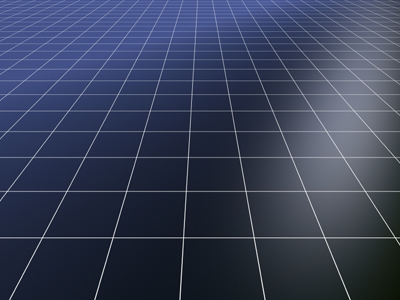On a grid, the x axis is horizontal.

# Position (Year 5)

In KS2 Maths as children advance to Year Five their knowledge of position and direction will increase. By now they should be very familiar with the points on a compass and the number of angles in a quarter, half or full rotation. Using this knowledge they should be able to work out angles positioned on a straight line or in a circle by taking away a given angle. They will now look at marking position using coordinates, the X axis and the Y axis on a grid.

One way we can mark positions is on a grid. We do this by using coordinates which are placed on an X axis and a Y axis. These coordinates will usually be written like this: (3,4) (3,6) (5,6) (5,4). Those particular ones would mark out a square. The first number represents a line on the X axis and the second number a line on the Y axis. Where the two lines meet is a particular point on the grid.

Test your positional skills by playing the following quiz.

1.
On a straight line, there is an angle of 72o. What is the other angle?
108o
208o
288o
98o
180o - 72o = 108o
2.
What is the name given to an angle that is less than 90o?
Acute
Obtuse
Right angle
Scalene
An acute angle can be thought of as the point of a sharp pencil
3.
How would you find the coordinates 2,3?
Start at 0,0 move 2 to the right and 3 up
Start at 0,0 move 3 to the right and 2 up
Start at 0,0 move 2 up and 3 to the right
Start at 0,0 move 3 up and 2 to the right
The first coordinate is always along the X axis
4.
On a grid, in which direction is the X axis?
Horizontal
Vertical
Diagonal
Curved
One way to remember this is the sound 'X' makes - it sounds a little bit like aCroSS and horizontal lines travel across the page
5.
What is the name of the vertical axis?
B
X
Y
Z
One way to remember this is that a 'Y' looks similar to a 'V' for Vertical
6.
What is the name given to an angle that is more than 90o and less than 180o?
Acute
Obtuse
Right angle
Scalene
An obtuse angle can be thought of as the point of a blunt pencil
7.
Which of these angles is obtuse?
90o
75o
150o
200o
200o is not obtuse as it is more than 180o
8.
How many degrees is a straight line?
360o
90o
45o
180o
A circle is 360o and a straight line is half a circle. 180o is half of 360o
9.
Two angles make a straight line. One is 25o; what is the other?
65o
335o
255o
155o
180o - 25o = 155o
10.
Which coordinates are the first on a grid?
1,1
0,0
0,1
1,0
0,0 is the place where the X axis and the Y axis cross one another